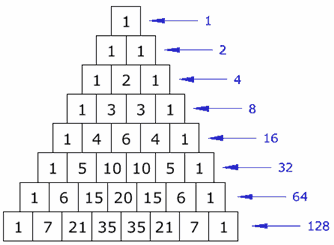# How do you use the binomial (2t-s)^5 using Pascal's triangle?

Jul 10, 2017

${\left(2 t - s\right)}^{5} = 32 {t}^{5} - 80 s {t}^{4} + 80 {s}^{2} {t}^{3} - 40 {t}^{2} {s}^{3} + 10 t {s}^{4} - {s}^{5}$

#### Explanation:

Pascal's triangle:The sixth row, which corresponds to a binomial raised to the fifth power, shows us the coefficient of each term of that expansion. So the first term has coefficient $1$, the second term $6$, etc.

We also know that, for a binomial raised to $n$, one nomial in each term will have decreasing exponents from $n$ to $0$ and the other nomial will have increasing exponents from $0$ to $n$.

$\therefore {\left(2 t - s\right)}^{5} = 1 {\left(2 t\right)}^{5} {\left(- s\right)}^{0} + 6 {\left(2 t\right)}^{4} {\left(- s\right)}^{1} + 10 {\left(2 t\right)}^{3} {\left(- s\right)}^{2} + 10 {\left(2 t\right)}^{2} {\left(- s\right)}^{3} + 6 {\left(2 t\right)}^{1} {\left(- s\right)}^{4} + 1 {\left(2 t\right)}^{0} {\left(- s\right)}^{5}$
$= 32 {t}^{5} - 80 s {t}^{4} + 80 {s}^{2} {t}^{3} - 40 {t}^{2} {s}^{3} + 10 t {s}^{4} - {s}^{5}$